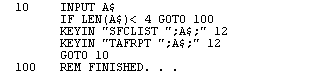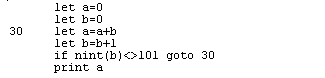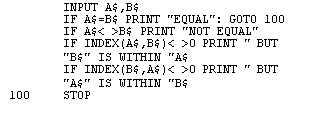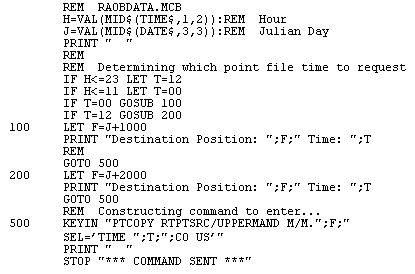McIDAS User's Guide
Version 2015.2

# McBASI Sample Programs

1. In this program, parameters are read from the RUN command until the list is exhausted. DEV=CNN suppresses the normal DONE messages from SFCLIST and TAFRPT. This sends the standard output to the CRT, but does not send the error or debug output. Use RUN KMSN KMKE KCLE KBOS KDCA or RUN KMEM KORD for this example. A step-by-step practice session with this example follows.2. This program illustrates a method of looping. The NINT function ensures that B will at some time be exactly equal to 101. No parameters are necessary in the RUN statement.3. In this example, the two character variables are read from the RUN statement. If they are identical, line 20 causes EQUAL to be displayed on the CRT and the program stops at line 100. If they are not equal, line 30 causes NOT EQUAL to be displayed, and lines 40 and 50 use the INDEX function to find out if one is a subset of the other. The semicolons between PRINT list items are omitted, as they are only required to prevent syntax ambiguities.

For example, RUN ABC ABC produces EQUAL on the CRT while RUN ABC DEFABCGHI produces NOT EQUAL plus BUT ABC IS WITHIN DEFABCGHI.4. This program copies the current day's 0 or 12 UTC mandatory RAOB MD file data to a workstation MD file. If the current hour is between 0 and 11, the 0 UTC RAOB data is copied to the dataset position equal to the value of the current three-digit Julian day plus 1000. If the current hour is between 12 and 23, the 12 UTC RAOB data is copied to the dataset position equal to the value of the current three-digit Julian day plus 2000.

If this program is run from the command scheduler every 12 hours for an entire year, the 0 UTC data for days 1 through 365 are placed in positions 1001 through 1365, and the 12 UTC data is placed in positions 2001 through 2365.The command RUN FILE=RAOBDATA.MCB run at 19:20 UTC on Julian day 98019 generates the output below.

 ```Destination Position: 2019 TIME: 12 PTCOPY RTPTSRC/UPPERMAND M/M.2019 SEL='TIME 12;CO US' *** COMMAND SENT *** ```